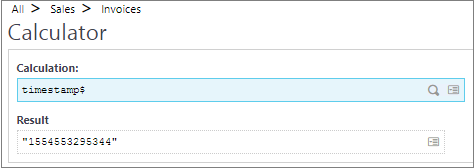# Timestamp\$ function in Sage X3

By | April 16, 2019

In Sage X3, We have function timestamp\$ which returns a string value containing a time stamp, which is the number of milliseconds elapsed since the first of January 1970, at 00:00:00 time GMT.

timestamp\$ returns a timestamp in ASCII format.Below is the snippet of example code provided by standard Sage X3.

<<Code snippet>>
Local decimal D
Local Decimal T : # Cannot be an integer because timestamp value is greater the 2^32
Local Integer T1
Local Char MY_TIME(8)
T=val(timestamp\$)
T1=mod(T,3600*24*1000)/1000 : # modulo the number of milliseconds in a day
MY_TIME=format\$(“N0:2″,int(T1/3600))+”:”+format\$(“N0:2″,int(mod(T1,3600)/60))+”:”+format\$(“N0:2”,mod(T1,60))
# Now, MY_TIME contains the GMT hour in hh:mm:ss format
<</Code snippet>>

Hope this blog helps!